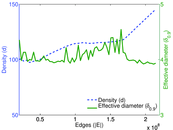# Delicious user–item

This network contains user–URL relations from http://delicious.com/. Left nodes represent users, right nodes represent URLs and an edge shows that a user tagged a URL.

 Code `Dui` Internal name `delicious-ui` Name Delicious user–item Data source http://dai-labor.de/IRML/datasets AvailabilityDataset is available for download Consistency checkDataset passed all tests Category Interaction network Node meaning User, URL Edge meaning Tag assignment Network formatBipartite, undirected Edge typeUnweighted, multiple edges Temporal dataEdges are annotated with timestamps Snapshot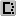Is a snapshot and likely to not contain all data

## Statistics

 Size n = 34,611,302 Left size n1 = 833,081 Right size n2 = 33,778,221 Volume m = 301,186,579 Unique edge count m̿ = 101,798,957 Wedge count s = 69,264,303,940 Claw count z = 108,117,582,742,195 Cross count x = 318,897,354,040,560,704 Maximum degree dmax = 143,470 Maximum left degree d1max = 143,470 Maximum right degree d2max = 100,625 Average degree d = 17.403 9 Average left degree d1 = 361.533 Average right degree d2 = 8.916 59 Fill p = 3.617 59 × 10−6 Average edge multiplicity m̃ = 2.958 64 Size of LCC N = 34,323,019 Diameter δ = 29 50-Percentile effective diameter δ0.5 = 5.093 45 90-Percentile effective diameter δ0.9 = 5.833 41 Mean distance δm = 5.143 67 Gini coefficient G = 0.879 437 Balanced inequality ratio P = 0.123 302 Left balanced inequality ratio P1 = 0.191 035 Right balanced inequality ratio P2 = 0.191 701 Relative edge distribution entropy Her = 0.842 841 Power law exponent γ = 3.649 70 Tail power law exponent γt = 1.761 00 Degree assortativity ρ = −0.031 510 6 Degree assortativity p-value pρ = 0.000 00 Spectral norm α = 3,694.59

## Plots

### Degree distribution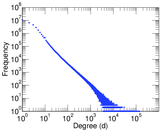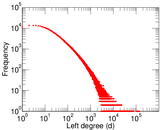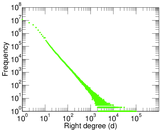### Cumulative degree distribution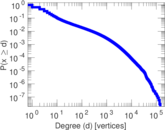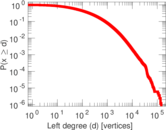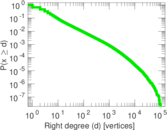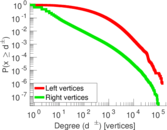### Lorenz curve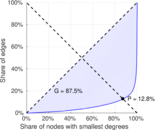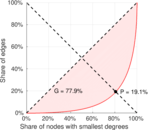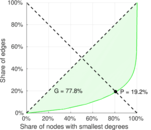### Spectral distribution of the adjacency matrix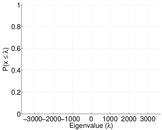### Spectral distribution of the normalized adjacency matrix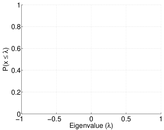### Spectral distribution of the Laplacian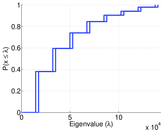### Spectral graph drawing based on the adjacency matrix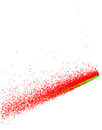### Spectral graph drawing based on the normalized adjacency matrix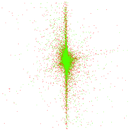### Degree assortativity### Hop distribution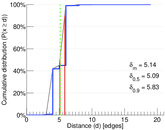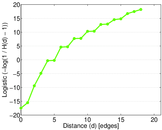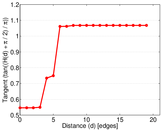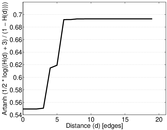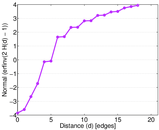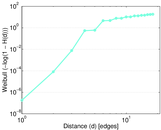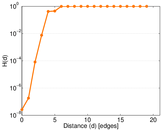### Edge weight/multiplicity distribution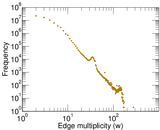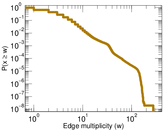### Temporal distribution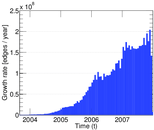### Diameter/density evolution